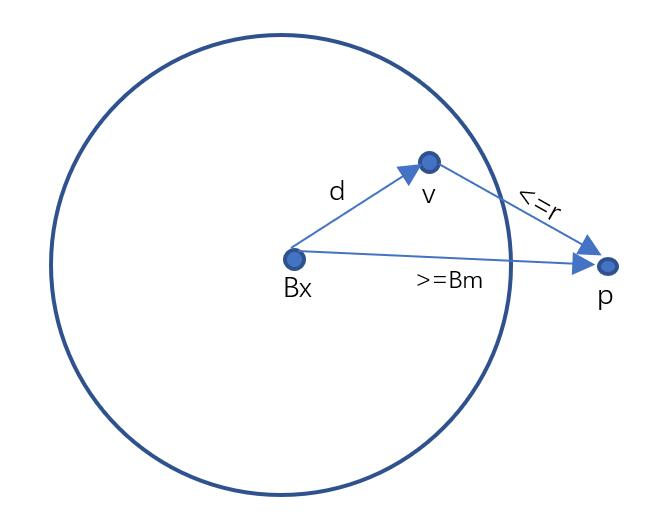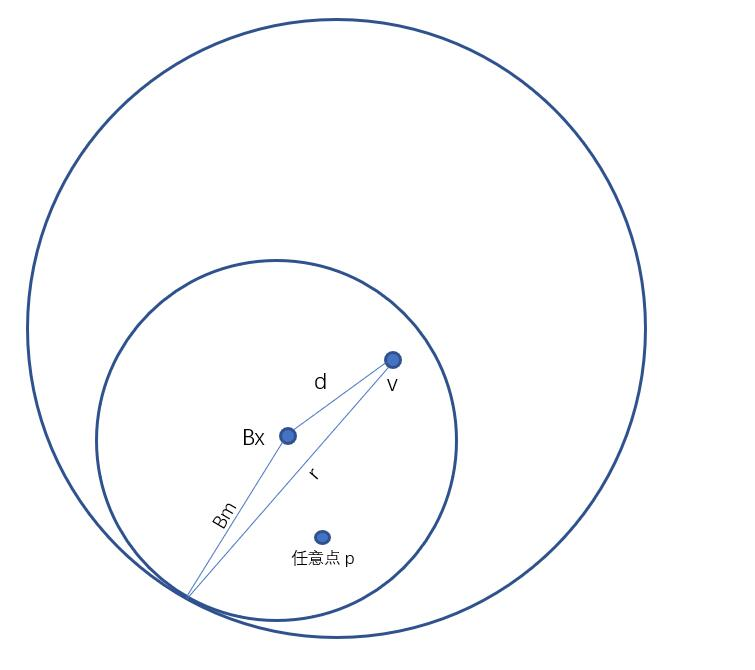# 如何用度量树实现通用的相似性查询

1. 球分解(Ball decomsitions)
度量距离D(X, Y)的定义
一个度量空间内的任何关系都应该满足下列条件：

d(x, y) = d(y, x)
0 < d(x, y), x != y
d(x, x) = 0
d(x, y) <= d(x, z) + d(z, y)

Bm = Bx与S中所有元素距离的中位数
Bleft = S中与除了Bx自身，与Bx距离小于等于中位数的元素组成的度量树
Bright = S中与Bx距离大于等于中位数的元素组成的度量树

(1)如果d(Bx, v) <= r, 那么将Bx加入所求点集 (2)如果d(Bx, v) + r >= Bm, 那么递归搜索右子树。当v与未知点p在空间中相对Bx位于同一侧的时候，v与p之间的距离是比较短的，换句话说就是更容易找到满足条件的点p。v与Bx三者如果满足（2）这条不等式，说明右子树中可能存在一个点与v距离小于r.三者之间构成的三角形关系如下图所示。(3)如果d(Bx, v) + Bm <= r, 那么左子树的所有点都加入所求点集。这条不等式很好理解，当它成立时，不论v相对于Bx在什么位置，b1都内含于b2，即b2都囊括了Bx左子树的所有点。1. 广义超平面分解(Generalized hyperplane decompositions)
可参考“超平面树 :度量空间中相似性搜索的索引结构”(李建中、张兆功)

GH-BASED树的插入函数